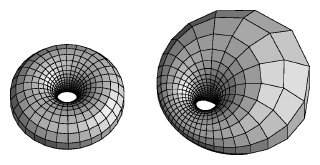The Mathematics of In- and Outside the Torus

Unlike the tori in "Torus Triptych", this is a projection of a torus lying originally in four-space, given parametrically by(cos q, sin q, cos f, sin f). Notice that this represents the cross product of two circles, one in the first two coordinates, and one in the second two. Note also that every point on this torus is at a distance of sqrt(2) from the origin, so the entire torus lies on the sphere of radius sqrt(2) in four-space. (In fact, this torus separates the sphere into two congruent solid tori.) Stereographic projection from the point (0,0,0,sqrt(2)) in four-space yields an image of the torus in three-space, and since stereographic projection maps circles to circles, the image of the torus given above under this map would be a torus of revolution. In contrast to the parameterization given for the "Torus Triptych", however, this one has the interesting property that it is a conformal mapping of the (q,f)-plane onto the torus of revolution.A torus projected from four-space can look like a torus of revolution (left). If part of it is closer to the projection point, part will appear larger, and it will form a cyclide of Dupin.